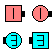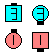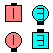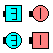Definitions of Square Dance Calls and Concepts

2 x 2 \$B\$+\$i!%(B

Leaders \$B\$O(B Box Circulate twice \$B\$r\$7\$F(B 1/4 In (Box Transfer) \$B\$r(B, Trailers \$B\$O(B Box Circulate - 1/4 In - Box Circulate \$B\$r\$7\$^\$9!%(B

2 x 2 \$B\$G=*\$o\$j\$^\$9!%(BSingle Checkmate\$B\$NA0(B \$B8e(BSingle Checkmate\$B\$NA0(B \$B8e(B

\$BCm
• \$B%*%j%8%J%k\$N(B Trailers \$B\$O(B Roll \$B\$G\$-\$^\$;\$s!%(B Single Checkmate \$B\$O(B Checkmate The Column [A2] \$B\$N(B Single \$B\$N%P!<%8%g%s\$G\$9!%(B Checkmate The Column \$B\$K\$D\$\$\$F\$b(B, Trailing two \$B\$O:G8e\$N%Q!<%H\$,(B Step Ahead \$B\$J\$N\$G(B, Roll \$B\$G\$-\$^\$;\$s!%(B
• Single Checkmate \$B\$O(B \$BNr;KE*\$K(B, each side \$B\$G9T\$&\$H\$-\$O(B Split Checkmate \$B\$H%3!<%k\$5\$l(B, center \$B\$"\$k\$\$\$O(B box \$B\$G9T\$&\$H\$-\$O(B Box Checkmate \$B\$H%3!<%k\$5\$l\$F\$-\$^\$7\$?!%(B
• Cheat: Box Counter Rotate 1/4 \$B\$r\$7\$F(B, Box Circulate \$B\$G\$9!%(B \$B\$3\$N(B cheat \$B\$O(B, \$B%*%j%8%J%k\$N(B Leaders \$B\$@\$1\$,(B Roll \$B\$G\$-\$k\$H\$\$\$&\$3\$H\$r\$O\$C\$-\$j\$5\$;\$^\$9!%B>\$N;qNA\$K\$O(B, \$BF1\$8\$h\$&\$J(B cheat (Box Circulate; Box Counter Rotate 1/4) \$B\$,\$"\$j\$^\$9\$,(B, \$B\$=\$l\$G\$OA40w\$,(B Roll \$B\$G\$-\$k\$h\$&\$K;W\$(\$F\$7\$^\$\$\$^\$9!%(B

Checkmate The Column [A2] (H.P. Williams 1975): Column \$B\$+\$i!%(B \$B;O\$a\$N(B 2 \$B?M\$O(B Circulate 4 spots \$B\$r\$7(B 1/4 In \$B\$r(B, \$B8e\$N(B2\$B?M\$O(B Circulate twice - 1/4 In - Circulate \$B\$r\$7\$^\$9!%(B \$BIaDL\$N(B Column \$B\$+\$i\$O(B, \$BJ?9T\$J(B Two-Faced Line \$B\$G=*\$o\$j\$^\$9!%(B

\$BSingle CONCEPT [C3A,C3B,C4].